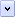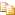You can expose properties in two ways:

• As simple values   The property is simply a global variable that can be read and written by the script component's user. Because the value is stored in a global value, you can manipulate it in your Windows® Script Component file's scripts.

• As functions   The property is defined using a function. This allows you to calculate a property value, and to control whether a property is read-only, read-write, or write-only.

You can also mark a property as the default value for a script component.

# To expose a property as a simple value

1. Create a <public> element as a child of the <component> element.

2. In the <public> element, include a <property> element that specifies the variable used to store property value.

3. To initialize the value of a simple property, create a global variable in the <script> element with a name matching propertyName (or propertyVariable, if you specified that) and assign it a value.

4. The following script component fragment illustrates two properties (name and tag) exposed as simple values. The properties are initialized using global variables in the <script> element.Note

A CDATA section is required to make the script in the <script> element opaque. For details, see Script Component Files and XML Conformance.Copy Code
```<public>
<property name="name"/>
<property name="tag" internalName="tagVar"/>
</public>

<script language="VBScript">
<![CDATA[
Dim name
name = "script component"   ' Initializes the value of name property.
Dim tagVar
tagVar = "10"   ' Initializes the value of tag property.
]]>
</script>```

# To expose a property using functions

1. In the script component file's <public> element, include a <property> element that contains a <get> element to define the read function and a <put> element to define the write function. If you do not include the <put> element, the property will be read-only. If you do not include the <get> element, the property will be write-only.

2. Write procedures in a <script> element outside the <public> element to implement the functions. The function for setting the property's value — the put function — must accept a single parameter, the value to set the property to.

The names of the procedures must match the internal names you specified in the <property> element. If you did not specify an internalName attribute, the names of the functions must be the name of the function with the get_ prefix for a read function, and with a put_ prefix for the write function.

3. For example, the following script component fragment is an example of a script component file that exposes three properties: sname, dateOfBirth, and age. The dateOfBirth property is defined by functions so it can include error checking. The age property is calculated, and is therefore defined as read-only.Note

A CDATA section is required to make the script in the <script> element opaque. For details, see Script Component Files and XML Conformance.Copy Code
```<public>
<property name="sname"/>
<property name="age">
</property>
<property name="dateOfBirth">
<put internalName="writeDOB"/>
</property>
</public>

<script language="VBScript">
<![CDATA[
Dim sname   ' Read-write sname property (no functions).
Dim gDOB   ' Global variable used to store date of birth.

' Gets value of dateOfBirth property.
End Function

Function writeDOB(newDOB)
' Sets value of dateOfBirth property.
If isDate(gDOB) Then
'Error checking
gDOB = newDOB
End If
End Function

' Calculates read-only age property.
If isDate(gDOB) Then
dobM = DatePart("m", gDOB)
dobD = DatePart("d", gDOB)
dobY = DatePart("yyyy", gDOB)
todayY = DatePart("yyyy", Date)
age = todayY - dobY

' Adjust if birthday has not yet occurred this year.
bday = DateValue(dobM & "/" & dobD & "/" & todayY)
If DateDiff("d", bday, DateValue(Date)) < 0 Then
age = age - 1
End If
End If
End Function
]]>
</script>```

You can specify a default property for a script component so the host application can get or set the property's value without explicitly naming the property. For example, if you have exposed a property called sname and marked it as the default, you can work with it in either of these ways in Visual Basic:Copy Code
```Set component = CreateObject("Component.MyComponent")
n = component.sname   ' Gets property explicitly.
n = component   ' Gets value of sname as default.```

To specify a default property, include an attribute assigning a special dispatch identifier (a dispid) to the method. For more information about dispids, see Exposing Events.

# To specify a default property

• In the <property> element, include the attribute dispid="0", as in the following example:Copy Code
`<property name="sname" dispid="0"/>`Note

This technique can be used to assign either a default property or a default method, but not both. There can be only one element in the script component with the dispid of zero.